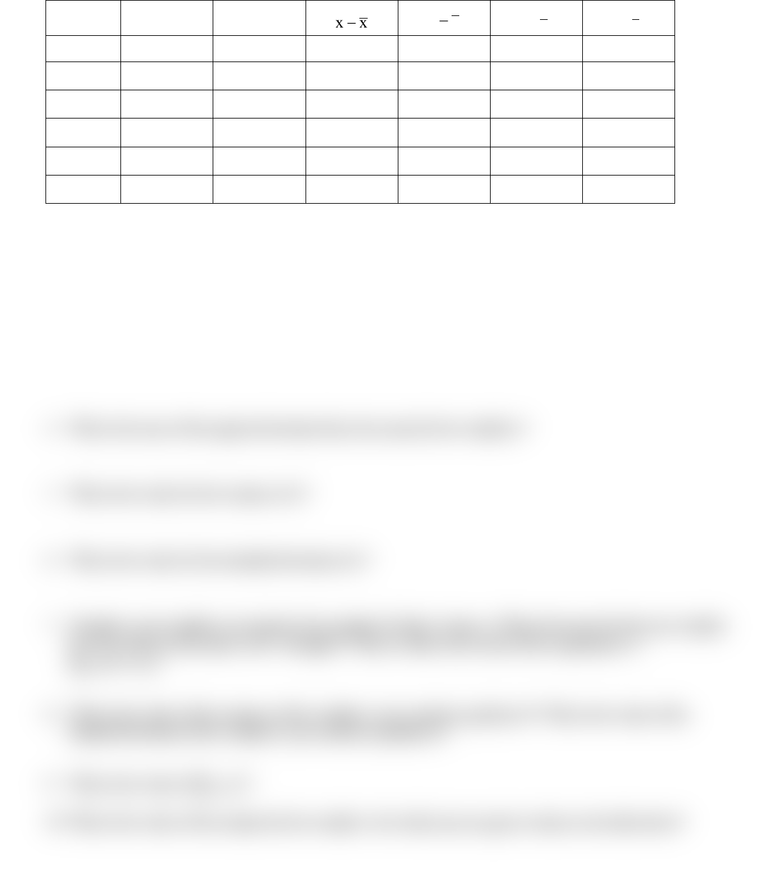Study Guides (390,000)
CA (150,000)
U of A (4,000)
STAT (100)
STAT141 (10)
Quiz

# STAT141 Quiz: Quiz 1 F2018 18,09,07aExam

Department
Statistics
Course Code
STAT141
Professor
Peter Hooper
Study Guide
Quiz

This preview shows half of the first page. to view the full 1 pages of the document.Quiz 1 questions, MGTSC 312, FALL 2018 to be answered this coming week in class, closed notes and book.
Also, for this quiz, you cannot use Excel tools or functions, or anything on your laptop but arithmetic features.
Getting into Chapter 4
You are given the following sample data:
Trip
km (x)
l (y)
xx
yy
2
)xx(
2
)yy(
col. 1
col. 2
col. 3
col. 4
col. 5
col. 6
1
500
60
2
200
25
3
700
80
4
600
75
1. Give the mean for x.
2. Fill in the values for column 3. What is the sum of the values you filled in for column 3?
3. Fill in the values for column 5.
4. What is the sum of the squared deviation from the mean for the variable x?
5. What is the value for the variance of x?
6. What is the value for the standard deviation of x?
7. Consider a new variable z set equal to the constant 10 plus 2 times x. What is the sum for this new variable
over the values of the index i for 1 through 4? That is, what is the value of the expression

 ?
8. What is the value of the variance of the variable z you created in problem 6? What is the value of the
standard deviation of the variable z you created in problem 6?
9. What is the value of
?
10. What is the value of the median for the variable x for which you are given values in the table above?